# Hacking Excel's Rank Function

Excel's RANK function (RANK.EQ) doesn't give a full ranking of data. It treats duplicates has having the same rank: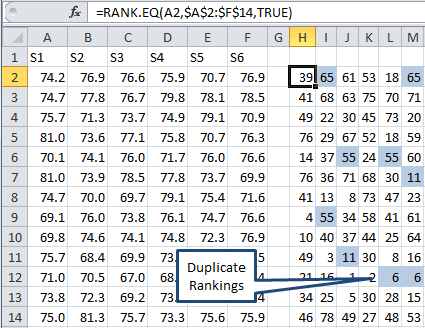Now, you could use RANK.AVG which would give 6.5 for both cells S12:T12, but it still isn't quite right.

### How To Hack the Rank Function in Tables

In Power Excel with MrExcel, Bill Jelen gave me the starting idea for how to tweak Excel's RANK function by counting how many times the value has already occurred and adding it to the rank. But I needed to do it for a table. Here's my solution:

1. The first cell can use RANK or RANK.EQ (Notice formula) to rank Cell A2 within A2:F15:2. The rest of the first row then needs to check for previous values:3. The rest of the first column has to check the previous rows:4. The rest of the cells need to check previous rows and cells: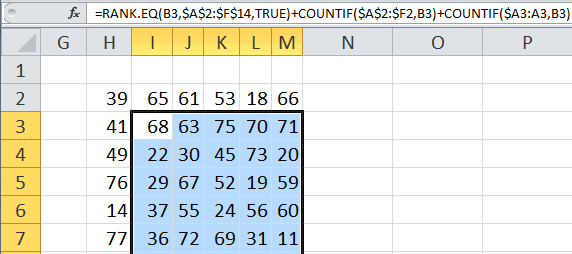Then you get a correct ranking of all values: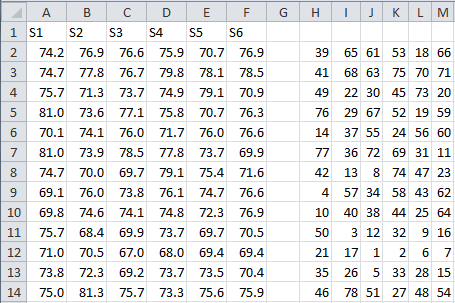### Here's My Point

Sometimes Excel Formulas have to be manipulated to provide the right starting point for analysis.
Sometimes you need a simple mathematical formula, sometimes you need something more exotic.

### Other Charts Included in QI Macros for Excel

Control Charts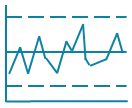Histograms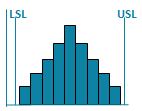Pareto ChartsBox PlotsFishbone Diagram